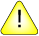This version of this document is no longer maintained. For the latest documentation, see http://www.qnx.com/developers/docs.

isinf(), isinff()

Test for infinity

Synopsis:

#include <math.h>

int isinf ( double x );

int isinff ( float x );

Arguments:

x
The number that you want to test.

Library:

libm

Use the -l m option to qcc to link against this library.

Description:

The isinf() and isinff() functions test to see if a number is "infinity."

Returns:

1
The value of x is infinity.
=/= 1
The value of x isn't infinity.

Examples:

#include <stdio.h>
#include <errno.h>
#include <inttypes.h>
#include <math.h>
#include <fpstatus.h>

int main(int argc, char** argv)
{
double a, b, c, d;

a = 2;
b = -0.5;
c = NAN;
d = 1.0/0.0;
printf("%f is %s \n", a,
(isinf(a)) ? "infinite" : "not infinite");
printf("%f is %s \n", b,
(isinf(b)) ? "infinite" : "not infinite");
printf("%f is %s \n", c,
(isinf(c)) ? "infinite" : "not infinite");
printf("%f is %s \n", d,
(isinf(d)) ? "infinite" : "not infinite");

return(0);
}

produces the output:

2.000000 is not infinite
-0.500000 is not infinite
NAN is not infinite
Inf is infinite

Classification:

isinf() is ANSI, POSIX 1003.1; isinff() is Unix

Safety:
Cancellation point No
Interrupt handler No
Signal handler No